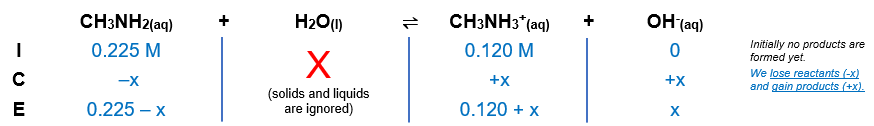# Problem: Solve an equilibrium problem (using an ICE table) to calculate the pH of each solution:a solution that is 0.225 M in CH3NH2 and 0.120 M in CH3NH3Br

###### FREE Expert Solution

CH3NH2weak base → proton acceptor
H2O → will act as the weak acid → proton donor

Equilibrium reaction:        CH3NH2(aq) + H2O(l)  CH3NH3+(aq) + OH-(aq)${\mathbf{K}}_{{\mathbf{b}}}\mathbf{=}\frac{\mathbf{products}}{\mathbf{reactants}}\phantom{\rule{0ex}{0ex}}{\mathbf{K}}_{\mathbf{b}}\mathbf{=}\frac{\left[{\mathrm{CH}}_{3}{\mathrm{NH}}_{3}{H}^{+}\right]\left[{\mathrm{OH}}^{-}\right]}{\left[{\mathrm{CH}}_{3}{\mathrm{NH}}_{2}\right]}$

Solids and liquids are not included in the expression

$\mathbf{4}\mathbf{.}\mathbf{4}\mathbf{×}{\mathbf{10}}^{\mathbf{-}\mathbf{4}}\mathbf{=}\frac{\left[0.120+x\right]\left[x\right]}{\left[0.225-x\right]}$

Now, we need to determine if we can remove (–x) from the equation. To do so, we need to determine the ratio of the initial concentration and Kb

null

$\mathbf{4}\mathbf{.}\mathbf{4}\mathbf{×}{\mathbf{10}}^{\mathbf{-}\mathbf{4}}\mathbf{=}\frac{\mathbf{\left[}\mathbf{0}\mathbf{.}\mathbf{120}\mathbf{+}\mathbf{x}\mathbf{\right]}\mathbf{\left[}\mathbf{x}\mathbf{\right]}}{\left[0.225-x\right]}\phantom{\rule{0ex}{0ex}}\mathbf{4}\mathbf{.}\mathbf{4}\mathbf{×}{\mathbf{10}}^{\mathbf{-}\mathbf{4}}\left(0.225-x\right)\mathbf{=}\frac{\mathbf{0}\mathbf{.}\mathbf{120}\mathbf{x}\mathbf{+}{\mathbf{x}}^{\mathbf{2}}}{\overline{)0.225-x}}\overline{)\left(0.225-x\right)}\phantom{\rule{0ex}{0ex}}\mathbf{9}\mathbf{.}\mathbf{9}\mathbf{×}{\mathbf{10}}^{\mathbf{-}\mathbf{5}}\mathbf{-}\mathbf{4}\mathbf{.}\mathbf{4}\mathbf{×}{\mathbf{10}}^{\mathbf{-}\mathbf{4}}\mathbf{x}\mathbf{=}\mathbf{0}\mathbf{.}\mathbf{120}\mathbf{x}\mathbf{+}{\mathbf{x}}^{\mathbf{2}}\phantom{\rule{0ex}{0ex}}\overline{)\mathbf{0}\mathbf{=}{\mathbf{x}}^{\mathbf{2}}\mathbf{+}\mathbf{0}\mathbf{.}\mathbf{12044}\mathbf{x}\mathbf{-}\mathbf{9}\mathbf{.}\mathbf{9}\mathbf{×}{\mathbf{10}}^{\mathbf{-}\mathbf{5}}}$

81% (132 ratings)###### Problem Details

Solve an equilibrium problem (using an ICE table) to calculate the pH of each solution:

a solution that is 0.225 M in CH3NH2 and 0.120 M in CH3NH3Br

Frequently Asked Questions

What scientific concept do you need to know in order to solve this problem?

Our tutors have indicated that to solve this problem you will need to apply the Buffer concept. You can view video lessons to learn Buffer. Or if you need more Buffer practice, you can also practice Buffer practice problems.